# Venn diagrams in Python and R

## What is Venn diagram?

• Sets of shapes especially circles to represent the logical relationships among two or more variables or datasets
• Venn diagrams are useful to identify the shared and unique elements belonging to each variable or datasets For example, in genomics experiments Venn diagrams are useful to identify the shared and unique genes among different conditions

## Create Venn diagrams using Python

Plot 2 and 3-way Venn diagrams using bioinfokit

``````>>>from bioinfokit import visuz
# 3-way Venn
>>>visuz.venn(vennset=(1,1,1,1,1,1,1))
# note: order the set in (100,010,110,001,101,011,111) format

# 2-way venn
>>>visuz.venn(vennset=(1,1,1))
# note: order the set in (111,100,010,001,110,101,011) format
``````

Generated Venn plots by above code,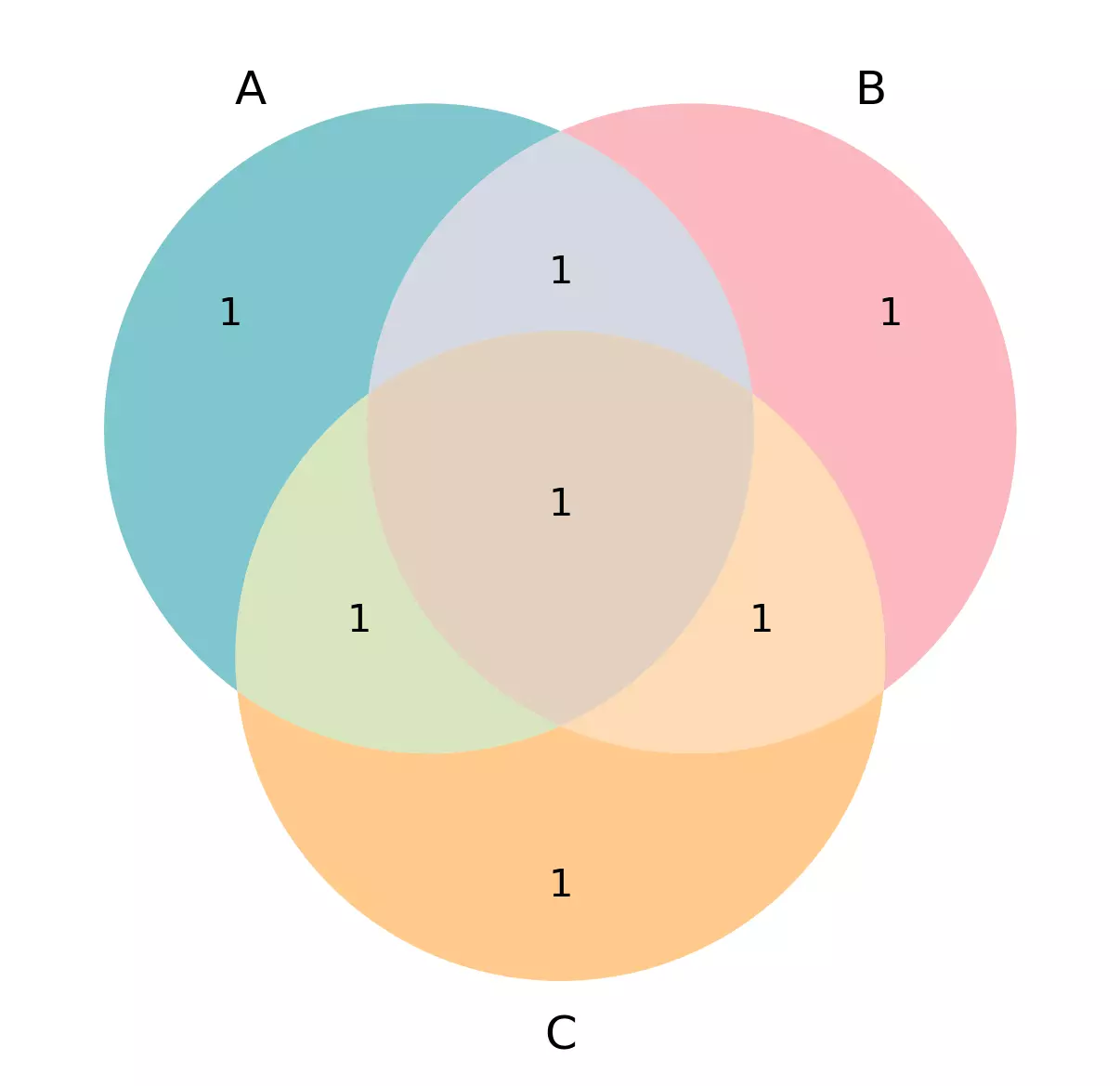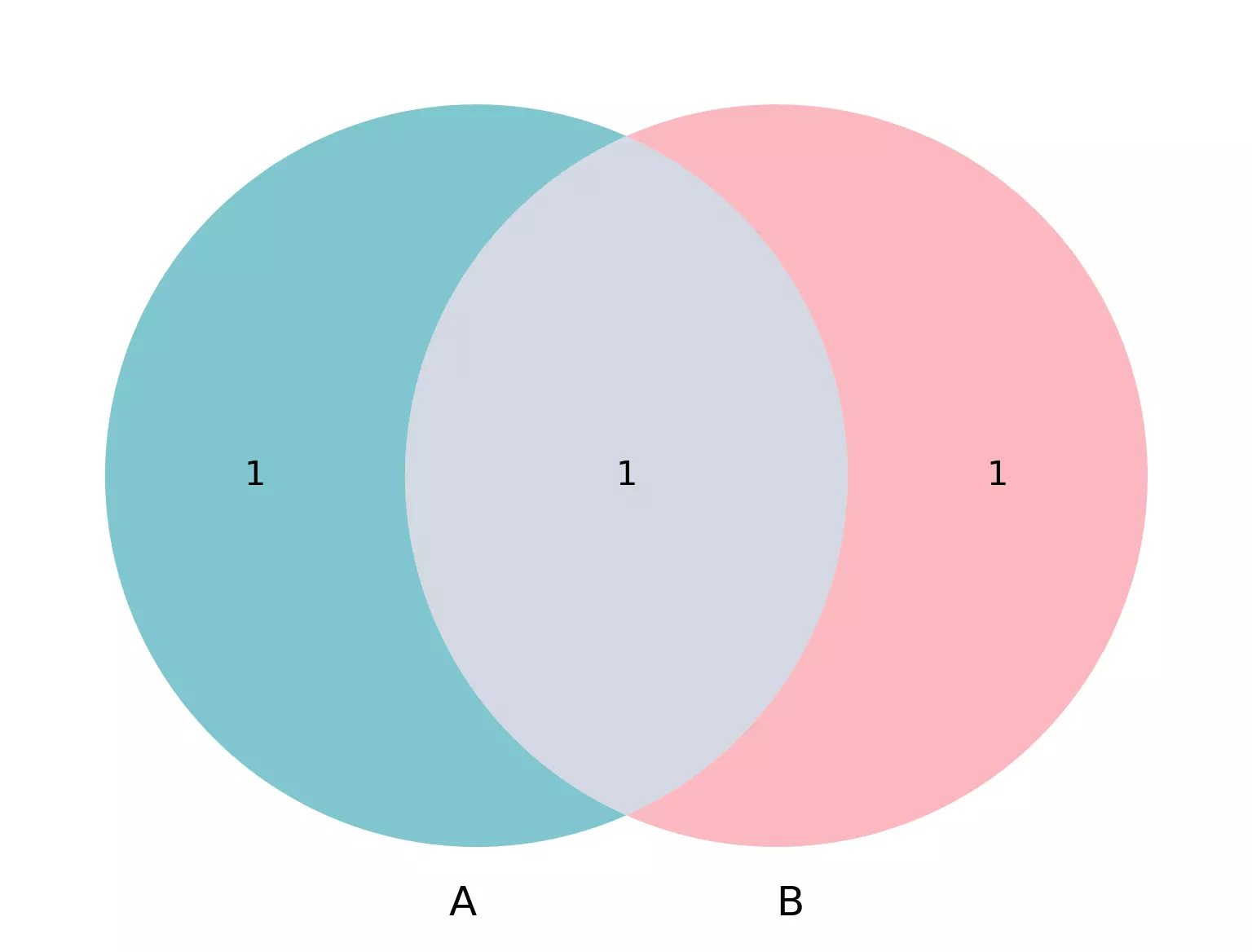With changes in default parameters for sets, and color, and labels

``````>>>visuz.venn(vennset=(300,600,402,470,200,230,408), vennlabel=("X", "Y", "Z"))
# note: order the set in (100,010,110,001,101,011,111) format

# change default color
# check for more colors at https://www.webucator.com/blog/2015/03/python-color-constants-module/
>>>visuz.venn(vennset=(300,600,402,470,200,230,408), vennlabel=("X", "Y", "Z"), venncolor=('#ff6347', '#008000', '#FF00FF'))
``````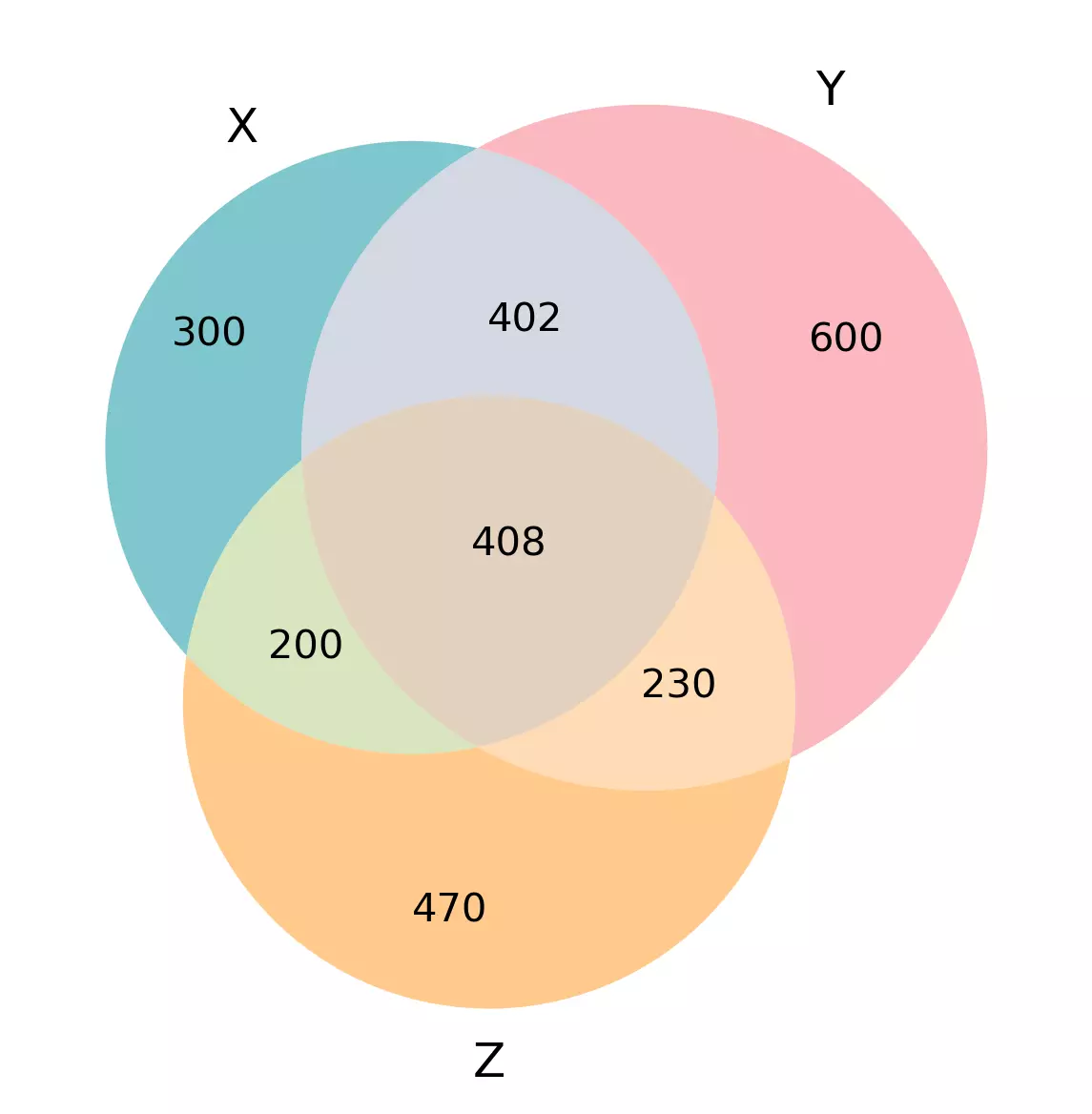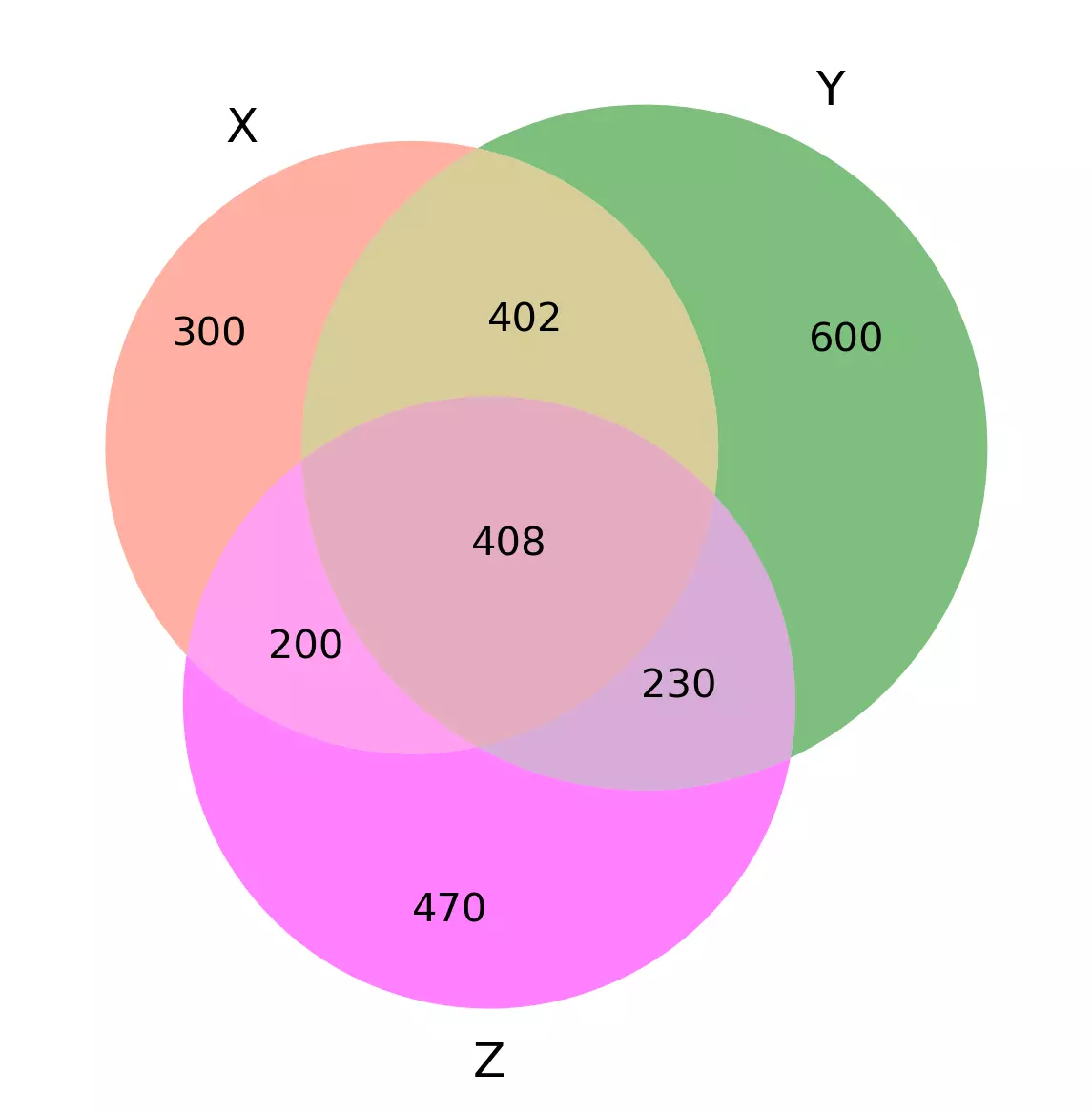With changes in default parameters for transparency

``````>>>visuz.venn(vennset=(300,600,402,470,200,230,408), vennlabel=("X", "Y", "Z"), vennalpha=0.9)
# note: order the set in (100,010,110,001,101,011,111) format

# 2-way venn
>>>visuz.venn(vennset=(300,600,402), vennlabel=("X", "Y"), venncolor=('#ff6347', '#008000'), vennalpha=0.9)
# note: order the set in (10,01,11) format
``````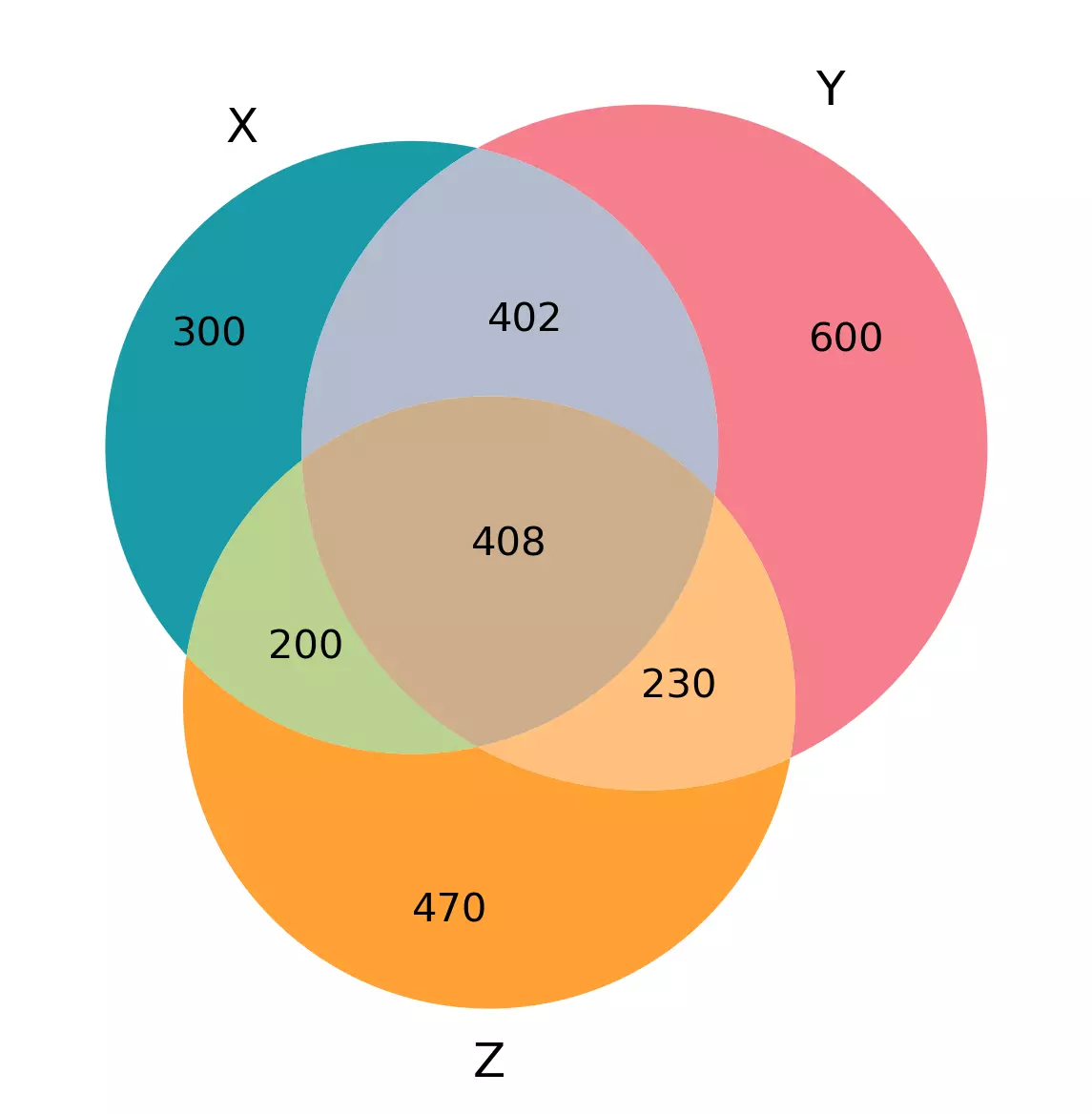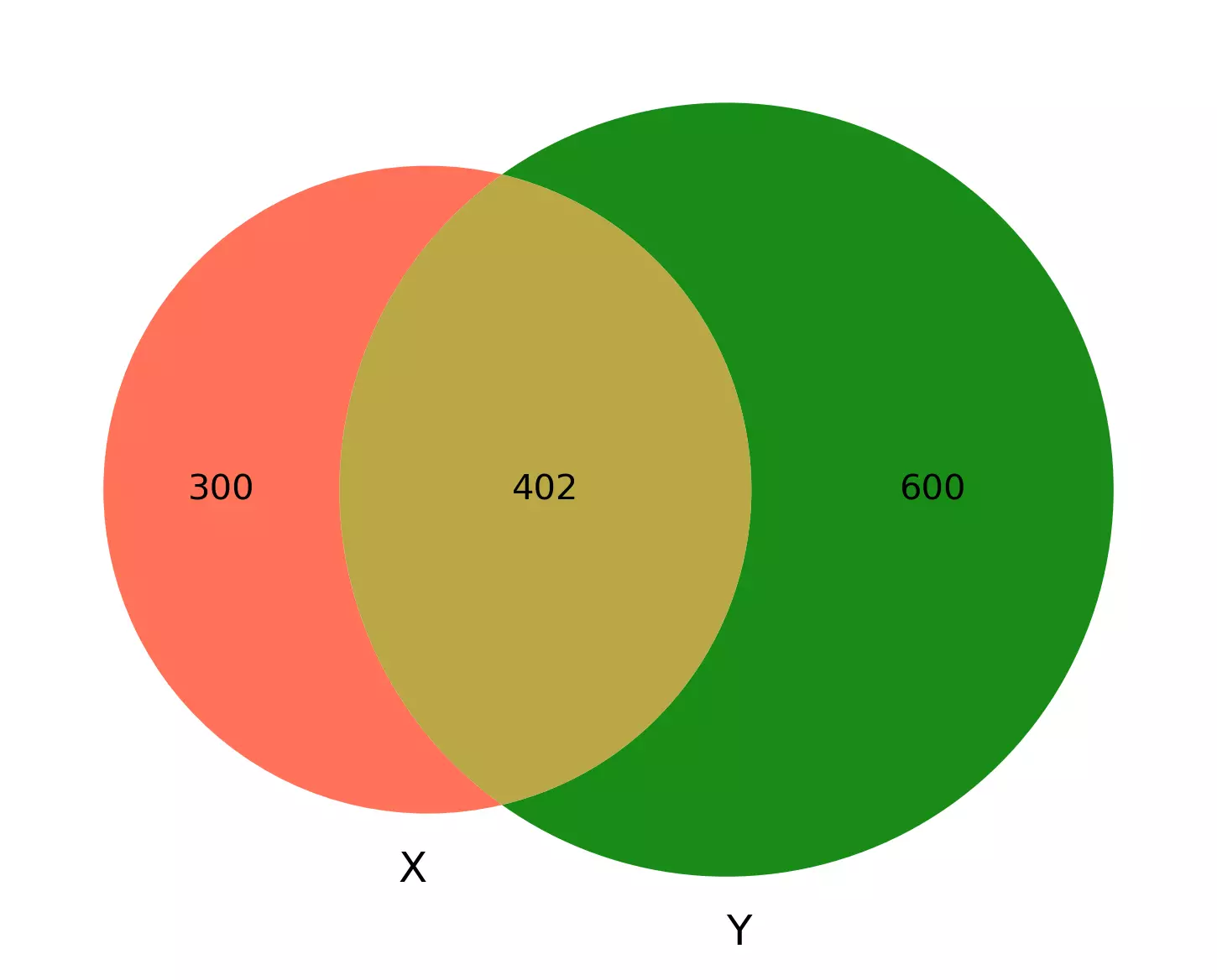## Create Venn diagrams using R

### Three-way Venn diagram

``````# install as install.packages('VennDiagram')
library(VennDiagram)
draw.triple.venn(area1=1310, area2=1640, area3=1308, n12=810, n23=638, n13=608,n123=408,
category=c('X', 'Y', 'Z'),  # label for each circle
fill=c('#00909e', '#f67280', '#ff971d'), # color for each circle
lwd=c(0,0,0)  # line width for each circle
)

``````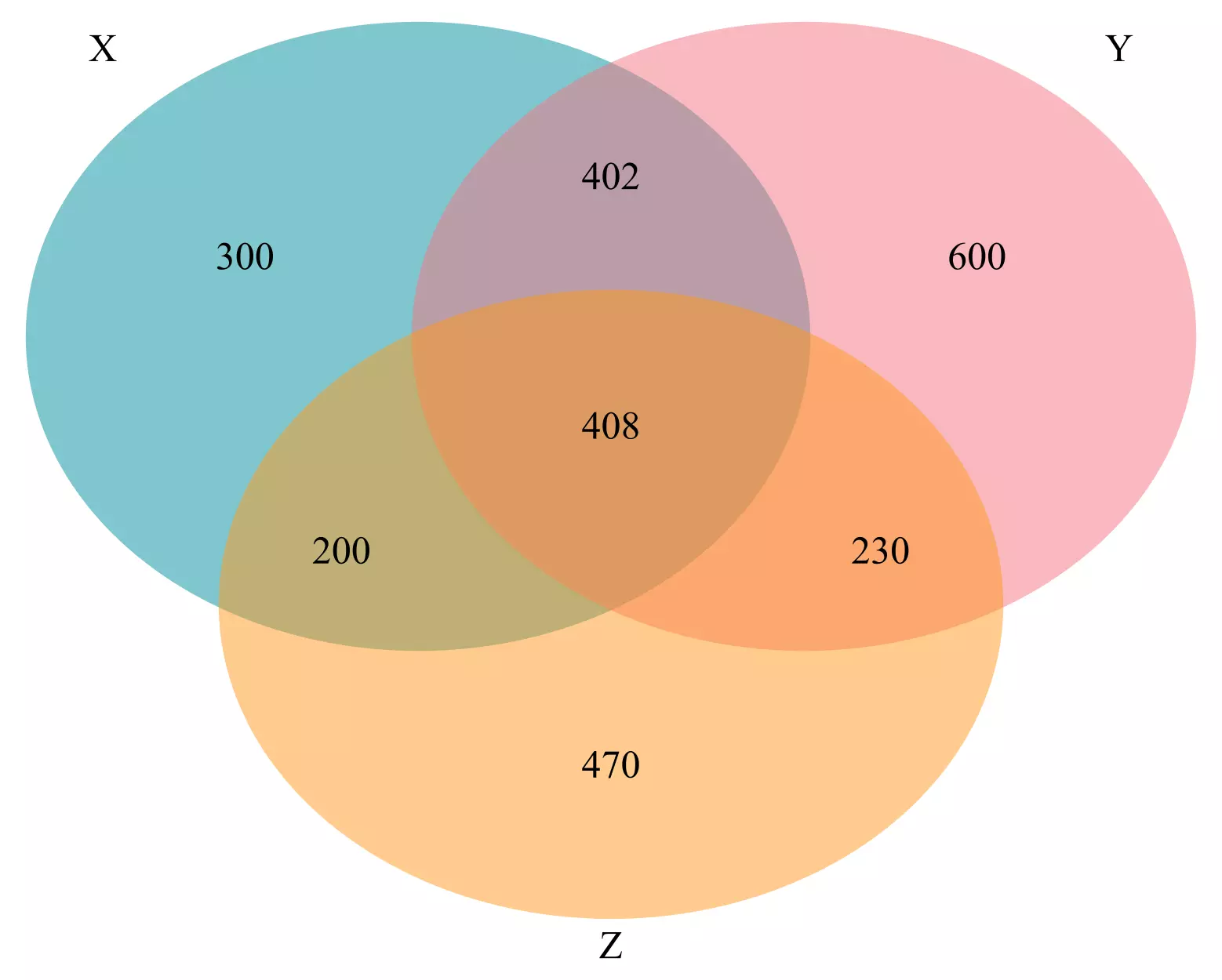### Two-way Venn diagram

``````draw.pairwise.venn(area1=100, area2=150, cross.area=50,
category=c('X', 'Y'),  # label for each circle
fill=c('#00909e', '#f67280'), # color for each circle
lwd=c(0,0)  # line width for each circle
)
``````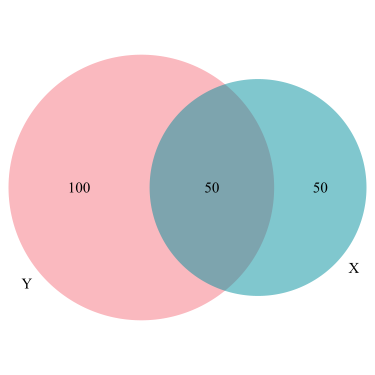## Subscribe to get new article to your email when published

* indicates required